# Finding the Mode and Range from a Line Plot Online Quiz

#### Master Mean : Learn the fundamentals of Mean Stack

59 Lectures 4 hours

#### Asp.Net Core & MERN MEAN Stack: Build Online Course and Shop

140 Lectures 38.5 hours

#### NodeJS Made Easy for MEAN or MERN Stack.

115 Lectures 6.5 hours

Following quiz provides Multiple Choice Questions (MCQs) related to Finding the Mode and Range from a Line Plot. You will have to read all the given answers and click over the correct answer. If you are not sure about the answer then you can check the answer using Show Answer button. You can use Next Quiz button to check new set of questions in the quiz.Q 1 - Find the mode and range from the following line plot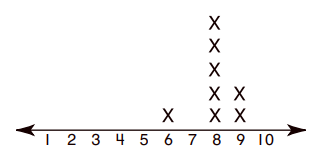### Explanation

Step 1:

The number that repeats most is 8; Mode = 8

Step 2:

Range = Max number – Min number

= 9 – 6 = 3

Step 3:

Mode = 8; Range = 3

Q 2 - Find the mode and range from the following line plot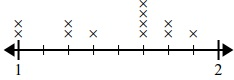### Explanation

Step 1:

The number that repeats most is 1$\frac{5}{8}$; Mode = 1$\frac{5}{8}$

Step 2:

Range = Max number – Min number

= 1$\frac{7}{8}$ – 1 = $\frac{7}{8}$

Step 3:

Mode = 1$\frac{5}{8}$; Range = $\frac{7}{8}$

Q 3 - Find the mode and range from the following line plot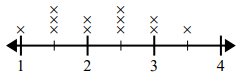### Explanation

Step 1:

The numbers that repeat most are 1.5, 2.5; Mode = 1.5, 2.5

Step 2:

Range = Max number – Min number

= 3.5 – 1 = 2.5

Step 3:

Mode = 1.5, 2.5; Range = 2.5

Q 4 - Find the mode and range from the following line plot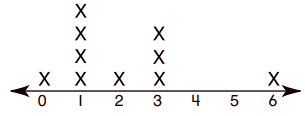### Explanation

Step 1:

The number that repeats most is 1; Mode = 1

Step 2:

Range = Max number – Min number

= 6 – 0 = 6

Step 3:

Mode = 1; Range = 6

Q 5 - Find the mode and range from the following line plot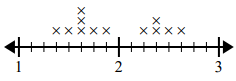### Explanation

Step 1:

The number that repeats most is 1$\frac{5}{8}$; Mode = 1$\frac{5}{8}$

Step 2:

Range = Max number – Min number

= 2$\frac{5}{8} \:–\: 1\frac{3}{8} = 1\frac{2}{8}$

Step 3:

Mode = 1$\frac{5}{8}$; Range = 1$\frac{2}{8}$

Q 6 - Find the mode and range from the following line plot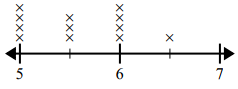### Explanation

Step 1:

The numbers that repeat most are 5, 6; Mode = 5, 6

Step 2:

Range = Max number – Min number

= 6.5 – 5 = 1.5

Step 3:

Mode = 5, 6; Range = 1.5

Q 7 - Find the mode and range from the following line plot### Explanation

Step 1:

The number that repeats most is 8; Mode = 8

Step 2:

Range = Max number – Min number

= 10 – 6 = 4

Step 3:

Mode = 8; Range = 4

Q 8 - Find the mode and range from the following line plot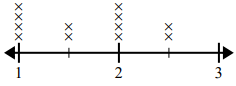### Explanation

Step 1:

The numbers that repeat most are 1, 2; Modes = 1, 2

Step 2:

Range = Max number – Min number

= 2.5 – 1 = 1.5

Step 3:

Mode = 1, 2; Range = 1.5

Q 9 - Find the mode and range from the following line plot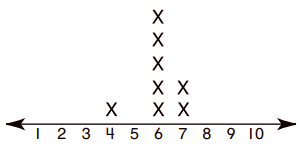### Explanation

Step 1:

The number that repeats most is 6; Mode = 6

Step 2:

Range = Max number – Min number

= 7 – 4 = 3

Step 3:

Mode = 6; Range = 3

Q 10 - Find the mode and range from the following line plot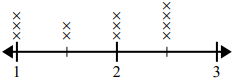### Explanation

Step 1:

The number that repeats most is 2.5; Mode = 2.5

Step 2:

Range = Max number – Min number

= 2.5 – 1 = 1.5

Step 3:

Mode = 2.5; Range = 1.5

finding_mode_and_range_from_line_plot.htm